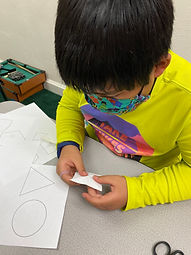Ms. Kylie

Target 1​

Lesson Type:

Review

Number Operation

:

Computation

Solve equations involving absolute value.

1:

Explain that the sums and differences of equations involving absolute value are found using distance and direction on a number line.

7th

Vocabulary:

Absolute Value, Variable

Activities:

-Students reviewed solving equations with absolute value

-They were given absolute value equations and solved the equations to find more than one solution. The students used their knowledge of isolating the variable to one side of the equation to find the possible solutions.Home Exploration

Guiding Questions:Absent Students:

Jude

Target 2

:

1:

Add whole numbers using the standard addition algorithm in fractional number problems.

2:

Explain the arithmetic process used for adding and subtracting fractions with like denominators.

3:

Recognize that only the numerators should be added or subtracted, not the denominators.

4:

Add fractions with like denominators, concluding that an improper fraction is likely the sum.

4th

Vocabulary:

Mixed Number, Denominator, Numerator

Activities:

-Students discussed what they knew about mixed numbers. They gave examples and drew pictures to model a mixed numbers.

-Students were given two mixed numbers with like denominators and added them together. They drew faction models to demonstrate their understanding of adding the mixed numbers.Home Exploration

Guiding Questions:Target 3

:

1:

Find multiple lines of symmetry in a figure.

2:

Draw lines of symmetry on given figures.

Define symmetry.

4th

Vocabulary:

Symmetry

Activities:

-Students were given several shapes to find multiple lines of symmetry.

-They folded each shape and drew the lines of symmetry. They recorded the amount of lines of symmetry and discussed what they discovered about lines of symmetry for each shape.Home Exploration